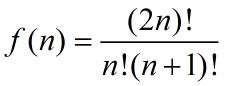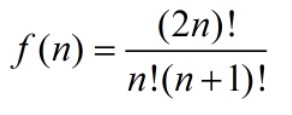• 对于有n个节点的二叉树
千次阅读
2021-01-25 23:24:20

1.n个节点的二叉树，最多可以有多少层？

A.n/2
B.log(n)
C.n-1
D.n
答案解析：
D
假设从根节点开始，根节点的层数为1，每一层一个节点，则有n层。数据结构 java
更多相关内容
• 对于n个节点二叉树，我们把这n个都当作父亲节点，一定可以补充（n+1）叶子节点，使之成为一棵（2n+1）个节点的完全二叉树。我们把原来的二叉树称作父亲树，即全是父亲节点的树。 一棵父亲树一定与一棵完全二叉树...
• ## n个节点的二叉树有多少种形态

千次阅读 多人点赞 2019-07-29 11:59:19
（1）先考虑只有一个节点的情形，设此时的形态f(1)种，那么很明显f(1)=1 （2）如果个节点呢？我们很自然想到，应该在f(1)的基础上考虑递推关系。那么，如果固定一个节点后，左右子树的分布情况为1=1+0=0+1，...

分析过程：
（1）先考虑只有一个节点的情形，设此时的形态有f(1)种，那么很明显f(1)=1

（2）如果有两个节点呢？我们很自然想到，应该在f(1)的基础上考虑递推关系。那么，如果固定一个节点后，左右子树的分布情况为1=1+0=0+1，故有f(2) = f(1) + f(1)

（3）如果有三个节点，（我们需要考虑固定两个节点的情况么？当然不，因为当节点数量大于等于2时，无论你如何固定，其形态必然有多种）我们考虑固定一个节点，即根节点。好的，按照这个思路，还剩2个节点，那么左右子树的分布情况为2=2+0=1+1=0+2。
所以有3个节点时，递归形式为f(3)=f(2) + f(1)*f(1) + f(2)。(注意这里的乘法，因为左右子树一起组成整棵树，根据排列组合里面的乘法原理即可得出)

（4）那么有n个节点呢？我们固定一个节点，那么左右子树的分布情况为n-1=n-1 + 0 = n-2 + 1 = … = 1 + n-2 = 0 + n-1。此时递归表达式为f(n) = f(n-1) + f(n-2)f(1) + f(n-3)f(2) + … + f(1)f(n-2) + f(n-1)

接下来我们定义没有节点的情况，此时也只有一种情况，即f(0)=1
那么则有:
f(0)=1，f(1)=1
f(2)=f(1)f(0)+f(0)f(1)
f(3)=f(2)f(0)+f(1)f(1)+f(0)f(2)

f(n)=f(n-1)f(0)+f(n-2)f(1)+……….+f(1)f(n-2)+f(0)f(n-1)
该数列称为卡特兰数（Catalan数），该递推关系的解为：即含n个节点的二叉树有f(n)种形态。

前几项为:  1, 1, 2, 5, 14, 42, 132,....

# 求n个节点的二叉树有多少种形态

class Solution:
def orderMultiply(self, x):
if x == 0:
return 1
else:
return x * self.orderMultiply(x-1)

def getStateofBinaryTree(self, n):
if n == 0:
return
elif n == 1:
return 1
else:
return self.orderMultiply(2*n) / self.orderMultiply(n) / self.orderMultiply(n+1)

n = 3
print(answer.getStateofBinaryTree(n))

## 【其他使用Catalan数解决的问题】

(1)矩阵链乘： P=a1×a2×a3×……×an，依据乘法结合律，不改变其顺序，只用括号表示成对的乘积，试问有几种括号化的方案？
(2)一个栈(无穷大)的进栈序列为1,2,3,..n,有多少个不同的出栈序列?
(3)有2n个人排成一行进入剧场。入场费5元。其中只有n个人有一张5元钞票，另外n人只有10元钞票，剧院无其它钞票，问有多少中方法使得只要有10元的人买票，售票处就有5元的钞票找零？(将持5元者到达视作将5元入栈，持10元者到达视作使栈中某5元出栈)
(4)将一个凸多边形区域分成三角形区域的方法数?
(5)在圆上选择2n个点,将这些点成对连接起来，使得所得到的n条线段不相交的方法数。
(6)一位大城市的律师在她住所以北n个街区和以东n个街区处工作。每天她走2n个街区去上班。如果她从不穿越（但可以碰到）从家到办公室的对角线，那么有多少条可能的道路？

转自 :

展开全文卡特兰数
• （1）先考虑只有一个节点的情形，设此时的形态f(1)种，那么很明显f(1)=1 （2）如果个节点呢？我们很自然想到，应该在f(1)的基础上考虑递推关系。那么，如果固定一个节点后，左右子树的分布情况为1=1+0=0+1，...

分析过程：
（1）先考虑只有一个节点的情形，设此时的形态有f(1)种，那么很明显f(1)=1

（2）如果有两个节点呢？我们很自然想到，应该在f(1)的基础上考虑递推关系。那么，如果固定一个节点后，左右子树的分布情况为1=1+0=0+1，故有f(2) = f(1) + f(1)

（3）如果有三个节点，（我们需要考虑固定两个节点的情况么？当然不，因为当节点数量大于等于2时，无论你如何固定，其形态必然有多种）我们考虑固定一个节点，即根节点。好的，按照这个思路，还剩2个节点，那么左右子树的分布情况为2=2+0=1+1=0+2。
所以有3个节点时，递归形式为f(3)=f(2) + f(1)*f(1) + f(2)。(注意这里的乘法，因为左右子树一起组成整棵树，根据排列组合里面的乘法原理即可得出)

（4）那么有n个节点呢？我们固定一个节点，那么左右子树的分布情况为n-1=n-1 + 0 = n-2 + 1 = … = 1 + n-2 = 0 + n-1。此时递归表达式为f(n) = f(n-1) + f(n-2)f(1) + f(n-3)f(2) + … + f(1)f(n-2) + f(n-1)

接下来我们定义没有节点的情况，此时也只有一种情况，即f(0)=1
那么则有:
f(0)=1，f(1)=1
f(2)=f(1)f(0)+f(0)f(1)
f(3)=f(2)f(0)+f(1)f(1)+f(0)f(2)
.
f(n)=f(n-1)f(0)+f(n-2)f(1)+……….+f(1)f(n-2)+f(0)f(n-1)
该数列称为卡特兰数（Catalan数），该递推关系的解为：![公式](https://img-blog.csdn.net/20150628170417849)

即含n个节点的二叉树有f(n)种形态。

## 【其他使用Catalan数解决的问题】

(1)矩阵链乘： P=a1×a2×a3×……×an，依据乘法结合律，不改变其顺序，只用括号表示成对的乘积，试问有几种括号化的方案？
(2)一个栈(无穷大)的进栈序列为1,2,3,..n,有多少个不同的出栈序列?
(3)有2n个人排成一行进入剧场。入场费5元。其中只有n个人有一张5元钞票，另外n人只有10元钞票，剧院无其它钞票，问有多少中方法使得只要有10元的人买票，售票处就有5元的钞票找零？(将持5元者到达视作将5元入栈，持10元者到达视作使栈中某5元出栈)
(4)将一个凸多边形区域分成三角形区域的方法数?
(5)在圆上选择2n个点,将这些点成对连接起来，使得所得到的n条线段不相交的方法数。
(6)一位大城市的律师在她住所以北n个街区和以东n个街区处工作。每天她走2n个街区去上班。如果她从不穿越（但可以碰到）从家到办公室的对角线，那么有多少条可能的道路？

展开全文算法
• =2）的二叉树有，求和的每一项，分别表示根的左子树为m个节点、右子树为 n-1-m个节点的情况。（总共n个节点，左子树m个节点，根节点有1，那么右子树的节点数为n-1-m） 刚好就是catalan数，直接用catalan数的公式...

记n个节点的二叉树形态个数为A[n]

1）0个节点的二叉树只有1种形态，A=0；1个节点的二叉树只有1种形态，A=1

2）n个节点（n>=2）的二叉树有，求和的每一项，分别表示根的左子树为m个节点、右子树为 n-1-m个节点的情况。（总共n个节点，左子树m个节点，根节点有1个，那么右子树的节点数为n-1-m个）

刚好就是catalan数，直接用catalan数的公式：h(n)==

展开全文• n个节点二叉树有多少种形态（Catalan数）】 分析过程： （1）先考虑只有一个节点的情形，设此时的形态f(1)种，那么很明显f(1)=1 （2）如果个节点呢？我们很自然想到，应该在f(1)的基础上考虑递推关系。...
• 一棵二叉树有n个结点。 该树满足任意结点的左子树结点个数和右子树的结点个数之差最多为1。 因为 2^n-1是表示n层满二叉树结点总数 所以 2^n-1>=二叉树结点总数 若结点为2022,则树的最大深度是? 2^10=...数据结构 b树
• 线索二叉树中为什么n个结点二叉树中，有n+1和空指针： 因为每结点有2空指针，而每度为1的结点有1空指针， 则总的空指针数为 2 * n0 + n1 而 n0 = n2 + 1 (二叉树的特性) 所以对上式化简，德总的空...数据结构
•java
• N个节点二叉树有多少种形态 这是一道阿里的面试题。其实算不上新鲜，但是我之前没关注过，如今碰到了，就顺便探讨下这问题吧：） 拿到这题，首先想到的是直接写出表达式肯定不行，所以必要从递推入手。...
• 证明：设完全二叉树的深度为h，则依据“深度为h的二叉树至多2h-1个结点（h≥1）”的性质，可得：2^(h-1)-1＜n ≤ 2^h-1 其等价于 2^(h-1)＜n +1 ≤ 2^h ，也即 2^(h-1) ≤ n^h ● 对式子 2^(h-1)＜n +1 ≤ 2^h 求...数据结构
• 递归算法计算n个结点二叉树的形态数 本题来自苏州大学872考研2016年真题。 设计递归算法，求出包涵n个结点的不同形态的二叉树的数目k。如：3个结点二叉树共有五种不同形态，即n=3时，k=5。 算法思想：本题与求...算法 数据结构 递归算法
• 没耐心的同学们可以直接拉到最底下看结论，兴趣的话可以浏览全篇文章 ...一棵完全二叉树具有1000个结点，则此完全二叉树有多少度为2的结点？ 完全二叉树699个节点，则叶子节点有多少？ 已知完全二叉树...
• n个结点构成的二叉树种类数 公式：C(2*n,n)/(n+1)
• n个无差别的节点构成的二叉树有多少种不同的结构？给定一整数n，请返回不同结构的二叉树的个数。保证结果在int范围内。测试样例：1返回：1class TreeCount {public: int Cmn(int m,int n) { if(m==n||n==0) ...
• <ol><li>一棵有nn>0）个结点的满二叉树共有____叶子和____非终端结点。</li></ol>人工智能 c++ c语言
• n个结点下，可构成多少种不同形态的二叉树 先来回顾下“树的定义”中的部分描述： 树是由唯一的根和若干棵互不相交的子树组成的。其中，每一棵子树又是一棵树，也是由唯一的根结点和若干颗互不相交的子树组成的。...数据结构
• 利用归纳法证明：一个有n个结点的非空二叉树的高度至少为 证明： 当n=1时，只有一个结点二叉树的高度为0，成立。 令x个结点二叉树的高度为h(x) 假设当n=k，k>=2时，结论也成立，即k个结点的非空...算法
•数据结构
• 高度为n的平衡二叉树最少需要多少个节点？高度为n的平衡二叉树最少需要多少个节点？参考 高度为n的平衡二叉树最少需要多少个节点？ 设f（n）为高度为n的平衡二叉树最少含有的节点数，则：f(1) = 1;f(2) = 2; f(3) = ...
• 严格二叉树，这定义就百度上有，维基百科都没查到。 严格二叉树的定义：如果一颗二叉树的每非终端节点都有且仅有两棵子树，则称这颗二叉树为严格二叉树。...首先证明一问题，对于有n个叶子的严格二叉树，必...
• n节点组成二叉树的个数 可以分析，当n=1时，只有1根节点，则只能组成1种形态的二叉树，令n节点可组成的二叉树数量表示为h(n)，则h(1)=1; h(0)=0;    当n=2时，1根节点固定，还有2-1节点...
• 具有3个结点二叉树有几种形态？ 正确答案: B 你的答案: B (正确) 4 5 6 7 解析 这是一道牛客网上的测试题，因为题目是求3个节点二叉树的形态，所以直接手画了。...
• 存在一棵总共2016个结点二叉树，其中16个结点只有一孩子 答案：F 分析： 假设没有孩子的结点（叶结点数为n₀，只有一孩子的结点（度为1的结点数为n₁，孩子的结点（度为2的结点数为n...数据结构 算法
• 如题，考研数据结构问题，具有n个结点且深度也为n二叉树有多少种？数据结构...